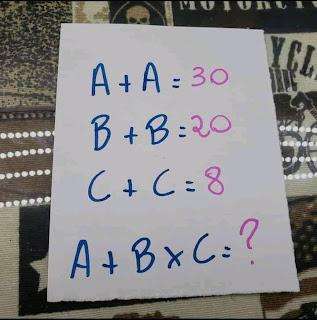0

# If A + A = 30, B+B = 20, C+C = 8, What's A+B ×C= ? answer# A+B ×C= ?

This tricky math quiz 'A + A = 30, B+B = 20, C+C = 8, What's A+B ×C= ? math quiz answer'  is making many people scratch their heads on social media especially on Facebook. Today I will show you how to solve the 'A+B × C = ?'  Math Quiz in detail.

To solve this kind of Tricky Math Quiz, one needs to apply Common sense plus Mathematics rules like BODMAS. Many people love brain games, dare games like Suduko, Puzzles, Sokoban, Quizzes and riddles. Be sure to share this one with friends. Also read: How many eggs can you see puzzle answer

Let's get started

## A + A = 30, B+B = 20, C+C = 8, A+B ×C= ?: How to solve

To get the correct answer, I will find the values of A, B and C then substitute in the final equation. The trick in the Quiz is in the final equation.

If A + A = 30,

Implied 2A = 30

A = 30 ÷ 2

A = 15.

If B + B = 20,

Implied B = 10

If C+ C = 8,

Implied C = 4.

Hence, A = 15, B = 10 and C = 4. I will substitute in the final equation.

## A + B × C =?

The correct answer will be 55 using BODMAS. That's

15 + 10 × 4 = ?

15 + 40= ? ( Applying BODMAS)

55 = ?.

Therefore, the correct answer to the Math Quiz  'If A + A = 30, B+B = 20, C+C = 8, What's A+B ×C= ?' is 55.

## Conclusion

Even though there are other methods to solve this tricky math quiz, BODMAS will give the correct answer. Some people Add before Multiplication which will give a wrong answer.

If you have different means of getting this answer or a different answer, Share with me in the comments section.

Also check

Tags